# Dividing fractions

## The division of the fraction a natural number

To divide a fraction by a natural number, we need the denominator to be multiplied by a number, and the numerator remain unchanged.

### Example of dividing fractions for a natural number

Example 1: Find the quotient of fractions in an integer (divide a fraction by a natural number):for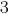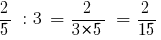Answer: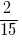Example 2: Find the quotient of fractions in an integer (divide a fraction by a natural number):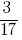for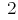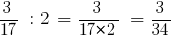Answer: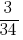## The division of a natural number into a fraction

To divide a natural number by a fraction, the number should be multiplied by a fraction the inverse of a given.

### An example of dividing a natural number into a fraction

Example 3: Find the quotient of positive integers to the fraction (divide the number on roll):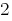and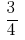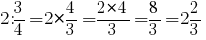Answer: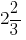### Dividing fractions

To divide one fraction by another, multiply the first fraction by the inverse of the second.

### Example of dividing fractions

Example 4: Find the quotient of one fraction to another (to separate the two fractions):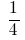and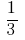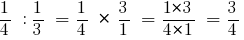Answer: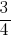Example 5: Find the quotient of one fraction to another (to separate the two fractions):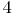and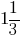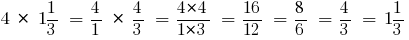Answer: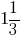## Division of mixed numbers

Todivide one mixed number to another, you need to:

• to convert mixed numbers to improper fractions;
• multiply the first fraction by the inverse of the second;
• reduce the resulting fraction;
• if you have received an improper fraction to turn an improper fraction into a mixed number.

### Examples of dividing mixed numbers

Example 6: Find the quotient of mixed fractions (divide two mixed fractions):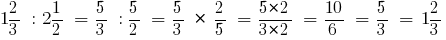Example 7: Find the quotient of mixed fractions (divide two mixed fractions):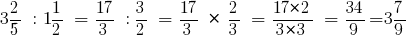## Dividing decimals

To divide a decimal fraction by a natural number, it is necessary:

• to divide a fraction by that number, ignoring the decimal point;
• to put a comma in private, when delenna a part.

## Division by a decimal

Division by decimals is replaced with division by a natural number.

To divide a number by a decimal, you should:

• 1 and divided by the divider to move the decimal point to the right on so many numbers, how many of them after the decimal point in the divisor;
• then perform a division by a natural number;
• if delena is not enough characters, right credited with zeros.

The rule is a consequence of the basic properties of the fraction (the line dividing the fraction model): the numerator and denominator of a fraction can be multiplied by a nonzero number (to expand a fraction).

In this case multiply to 10,100,1000, etc.

For example,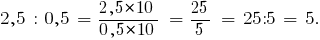In short can be written as: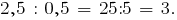Moved someone in divided by 2.5 and the divider 0.5 to so many characters, how many of them after the decimal point in the divisor is 0.5, that is, one character.

### Dividing decimals by 0,1; 0,01; 0,001, etc.

To divide decimals by 0,1; 0,01; 0,001 , etc., necessary

• to move to this point as many figures to the right, how many zeros comes before one in the divisor (or multiply the dividend and the divisor by 10, 100, 1000і etc.)
• If digits is not enough, first you have to ascribe at the end of a decimal, the zeros (as needed).

Consider the examples of dividing by 0,1; 0,01; 0,001, by applying the rule of division by a decimal fraction:

1. in divided by the divisor and move the decimal to the right by as many digits
how many of them after the decimal point in the divisor;
2. then perform the division by a natural number.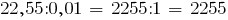Tags:
Chapter:
Versions in other languages:
Share with friends: